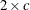### Comparing Two Independent Samples

SAS/STAT software provides several nonparametric tests for location and scale differences for two independent samples.

When you perform these tests, your data should consist of a random sample of observations from two different populations. Your goal is to compare either the location parameters (medians) or the scale parameters of the two populations. For example, suppose your data consist of the number of days in the hospital for two groups of patients: those who received a standard surgical procedure and those who received a new, experimental surgical procedure. These patients are a random sample from the population of patients who have received the two types of surgery. Your goal is to decide whether the median hospital stays differ for the two populations.

#### Tests in the NPAR1WAY Procedure

The NPAR1WAY procedure provides the following location tests: Wilcoxon rank sum test (Mann-Whitney U test), median test, Savage test, and Van der Waerden (normal scores) test. Note that the Wilcoxon rank sum test can also be obtained from the FREQ procedure. PROC NPAR1WAY provides Hodges-Lehmann estimation of the location shift between two samples, including asymptotic (Moses) and exact confidence limits.

In addition, PROC NPAR1WAY produces the following tests for scale differences: Siegel-Tukey test, Ansari-Bradley test, Klotz test, and Mood test. PROC NPAR1WAY also provides the Conover test, which can be used to test for differences in both location and scale.

Additionally, PROC NPAR1WAY provides tests that use the input data observations as scores, enabling you to produce a wide variety of tests. You can construct any scores for your data with the DATA step, and then PROC NPAR1WAY computes the corresponding linear rank test. You can directly analyze the raw data this way, producing the permutation test known as Pitman’s test.

When data are sparse, skewed, or heavily tied, the usual asymptotic tests might not be appropriate. In these situations, exact tests might be suitable for analyzing your data. The NPAR1WAY procedure can produce exact p-values for all of the two-sample tests for location and scale differences.

See Chapter 65: The NPAR1WAY Procedure, for details, formulas, and examples of these tests.

#### Tests in the FREQ Procedure

The FREQ procedure provides nonparametric tests that compare the location of two groups and that test for independence between two variables.

The situation in which you want to compare the location of two groups of observations corresponds to a table with two rows. In this case, the asymptotic Wilcoxon rank sum test can be obtained by using SCORES=RANK in the TABLES statement and by looking at either of the following:

• the Mantel-Haenszel statistic in the list of tests for no association. This is labeled as Mantel Haenszel Chi-Square, and PROC FREQ displays the statistic, the degrees of freedom, and the p-value. To obtain this statistic, specify the CHISQ option in the TABLES statement.

• the CMH statistic 2 in the section on Cochran-Mantel-Haenszel statistics. PROC FREQ displays the statistic, the degrees of freedom, and the p-value. To obtain this statistic, specify the CMH2 option in the TABLES statement.

When you test for independence, the question being answered is whether the two variables of interest are related in some way. For example, you might want to know if student scores on a standard test are related to whether students attended a public or private school. One way to think of this situation is to consider the data as a two-way table; the hypothesis of interest is whether the rows and columns are independent. In the preceding example, the groups of students would form the two rows, and the scores would form the columns. The special case of a two-category response (Pass/Fail) leads to atable; the case of more than two categories for the response (A/B/C/D/F) leads to atable, where c is the number of response categories.

For testing whether two variables are independent, PROC FREQ provides Fisher’s exact test. For atable, PROC FREQ automatically provides Fisher’s exact test when you specify the CHISQ option in the TABLES statement. For atable, use the FISHER option in the EXACT statement to obtain the test.

See Chapter 38: The FREQ Procedure, for details, formulas, and examples of these tests.